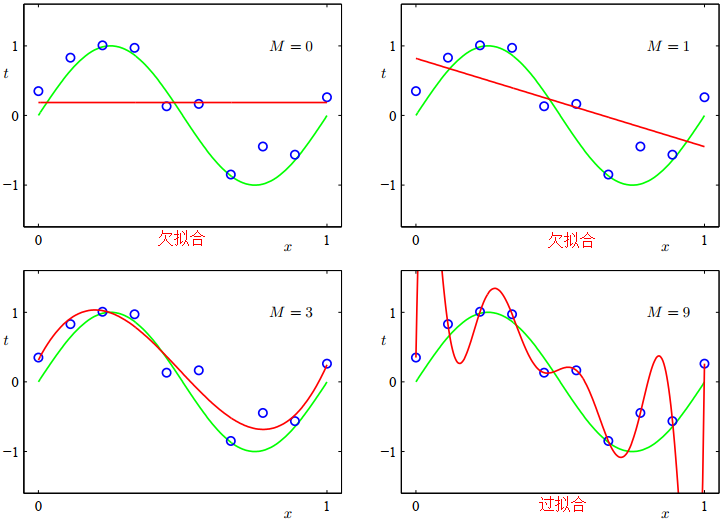V1

2022/07/17阅读：26主题：默认主题

# 标准化、归一化和正则化的关系

首先，标准化的英文是Standardization，归一化的英文是Normalization，正则化的英文是Regularization。标准化是特征缩放的一种方式，需要注意的是标准化之后的数据分布并不一定是正态分布，因为标准化并不会改变原始数据的分布。归一化的目的是消除不同量纲及单位影响，提高数据间的可比性。正则化的目的是为了防止过拟合。文中涉及代码下载参考。

# 一.标准化

## 3.标准化实现

from sklearn import preprocessingimport numpy as npX_train = np.array([[1., -1., 2.], [2., 0., 0.], [0., 1., -1.]])X_train_result = preprocessing.scale(X_train, axis=0) #axis=0表示按列进行标准化print(X_train_result)print(X_train_result.mean(axis=0)) #均值为0print(X_train_result.std(axis=0))  #标准差为1

[[ 0.         -1.22474487  1.33630621] [ 1.22474487  0.         -0.26726124] [-1.22474487  1.22474487 -1.06904497]][0. 0. 0.][1. 1. 1.]

from sklearn import preprocessingimport numpy as npX_train = np.array([[1., -1., 2.], [2., 0., 0.], [0., 1., -1.]])scaler = preprocessing.StandardScaler().fit(X_train)X_scaled = scaler.transform(X_train)print(X_scaled.mean(axis=0)) #均值为0print(X_scaled.std(axis=0))  #标准差为1X_test = [[-1., 1., 0.]]     #使用训练集的缩放标准来标准化测试集，这里的缩放标准指的就是训练集的列的均值和标准差print(scaler.transform(X_test))

[0. 0. 0.][1. 1. 1.][[-2.44948974  1.22474487 -0.26726124]]

# 二.归一化

## 1.Min-Max Normalization

import numpy as npfrom sklearn import preprocessing as ppX_train = np.array([[ 1., -5., 8.], [ 2., -3., 0.], [ 0., -1., 1.]])scaler = pp.MinMaxScaler().fit(X_train) #默认数据压缩范围为[0,1]print(scaler.transform(X_train))

[[0.5   0.    1.   ] [1.    0.5   0.   ] [0.    1.    0.125]]

## 2.Mean Normalization

import numpy as npfrom sklearn import preprocessing as ppX_train = np.array([[ 1., -5., 8.], [ 2., -3., 0.], [ 0., -1., 1.]])scaler = pp.MinMaxScaler(feature_range=(-1, 1)) #设置数据压缩范围为[-1,1]scaler = scaler.fit(X_train)print(scaler.transform(X_train))

[[ 0.   -1.    1.  ] [ 1.    0.   -1.  ] [-1.    1.   -0.75]]

# 三.正则化

简单理解使用正则化的目的就是为了防止过拟合，当然还有其它防止过拟合的方法，比如降低特征维度。先举个例子说下为什么降低特征维度也可以防止过拟合，然后再说明正则化是如何防止过拟合的。首先要搞明白过拟合的本质是什么？就是把噪音也当做事物的特征进行了建模。假如一只小鸟受伤了，暂时不会飞翔，在构建鸟类分类器的时候，把能否飞翔这个噪音也学习成模型的特征了，这样正常的能够飞翔的小鸟就判断为不是鸟类了，当然这是一个过拟合的很牵强的例子，但是也可说明一定的问题。正则化是如何防止过拟合的呢？

正则化方法是一个常量，它通过限制模型的复杂度，使得复杂的模型能够在有限大小的数据集上进行训练，而不会产生严重的过拟合。正则项越小，惩罚力度越小，极端情况正则项为0时，就会造成过拟合问题；正则化越大，惩罚力度越大，就会容易出现欠拟合问题。通过上图可以直观的看到采用L1范数时平方误差项等值线与正则化项等值线的交点出现在坐标轴上，即 或者 。当采用L2范数时，两者的交叉点常出现在某个象限中，即 。这样采用L1正则化比L2正则化更容易得到稀疏解。L2正则化优势是处处可导，L1正则化有拐点，不是处处可微，但可以得到更加稀疏的解。

Preprocessing data：https://scikit-learn.org/stable/modules/preprocessing.html
机器学习中常常提到的正则化到底是什么意思：https://www.zhihu.com/question/20924039
模式识别和机器学习[中文版]：https://url39.ctfile.com/f/2501739-616549609-5fe8d7?p=2096 (访问密码: 2096)
模式识别与机器学习[英文版]：https://url39.ctfile.com/f/2501739-616549614-9897ab?p=2096 (访问密码: 2096)
标准化、归一化和正则化的关系.py：https://url39.ctfile.com/f/2501739-616549789-16fc6a?p=2096 (访问密码: 2096)V1